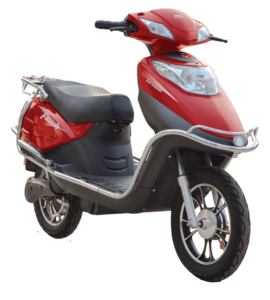# Explanation of Ah, kW and kWh in an Electric Vehicle

If you ever observe an electric vehicle specification list , kW, kWh and Ah are very confused unless you are an electrical student. Usually these technical specs can be used to pick the best of any two EVs or to calculate the performance an electric vehicle .This article helps you in understanding deeply about what is Ah, kW and kWh in an electric Vehicle. Lets get started.

## Explanation of Ah, kW and kWh in an Electric Vehicle

In general petrol run vehicles, we know that 1 liter of petrol can give a certain mileage ,but if we consider electric vehicles, the mileage is calculated in kWh.

# What is kWh in an Electric Vehicle

## General Terminology to Understand

In general, for any work to do, you need some power, and the power is measured in watt(W). Any electric vehicle battery specification is given in terms of V | Ah.  We can convert into watts, by simply multiplying V*Ah = Watt hour value.

## What is KWH ?

K means KILO ; KILO = 1000

W means Watt, which is the unit of power

H means Hour

kWh means KILO WATT HOUR.

## I’m considering Hero Electric Flash Scooter Specifications as an example to explain all the terms

### Hero Electric Flash Specifications• Battery power = 48V | 28Ah = 48 * 28  =1344 Watt Hour
• Range is 60 km
• Motor Power = 550 Watts
• Charging Time : 4 hours

## What is kWh in an Electric Vehicle ?

kWh is an electric vehicle battery storage capacity in hours.

Example: 1344 Watt hour means 1.344 kWh, which is the battery storage capacity of Hero Electric Flash.

# What is kW in an Electric Vehicle

kW is the power of an electric vehicle motor require to run the vehicle.

550 Watts Hour is the motor power in Hero Electric Flash = 0.55 Kilo Watts Hour (Converted into Kilo Watts).

0.55 kW is the Motor power require for an Hour.

If you run Electric Flash faster, then the power consuming by the motor is high, and it will consume more power and vise versa.

kW is the amount of power your motor consuming to run an electric vehicle.

## Ah in an Electric Vehicle

Ampere is the unit for electrical Current,  while charging the amount of current stored in a battery is denoted as Amperes. Ah means “Ampere Hour“, for Hero Electric Flash 28Ah battery  , 28 Ampere current can produce in one hour or 1 Ampere current can be produced  in 28 hours depends on the motor load requirement.

The more Ah value , the more battery capacity an electric vehicle can get.